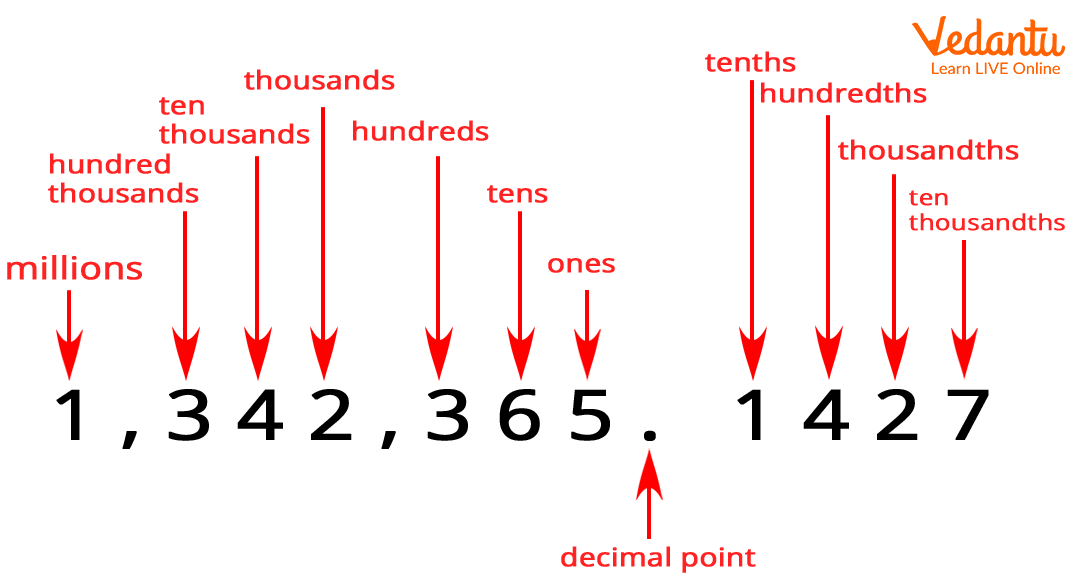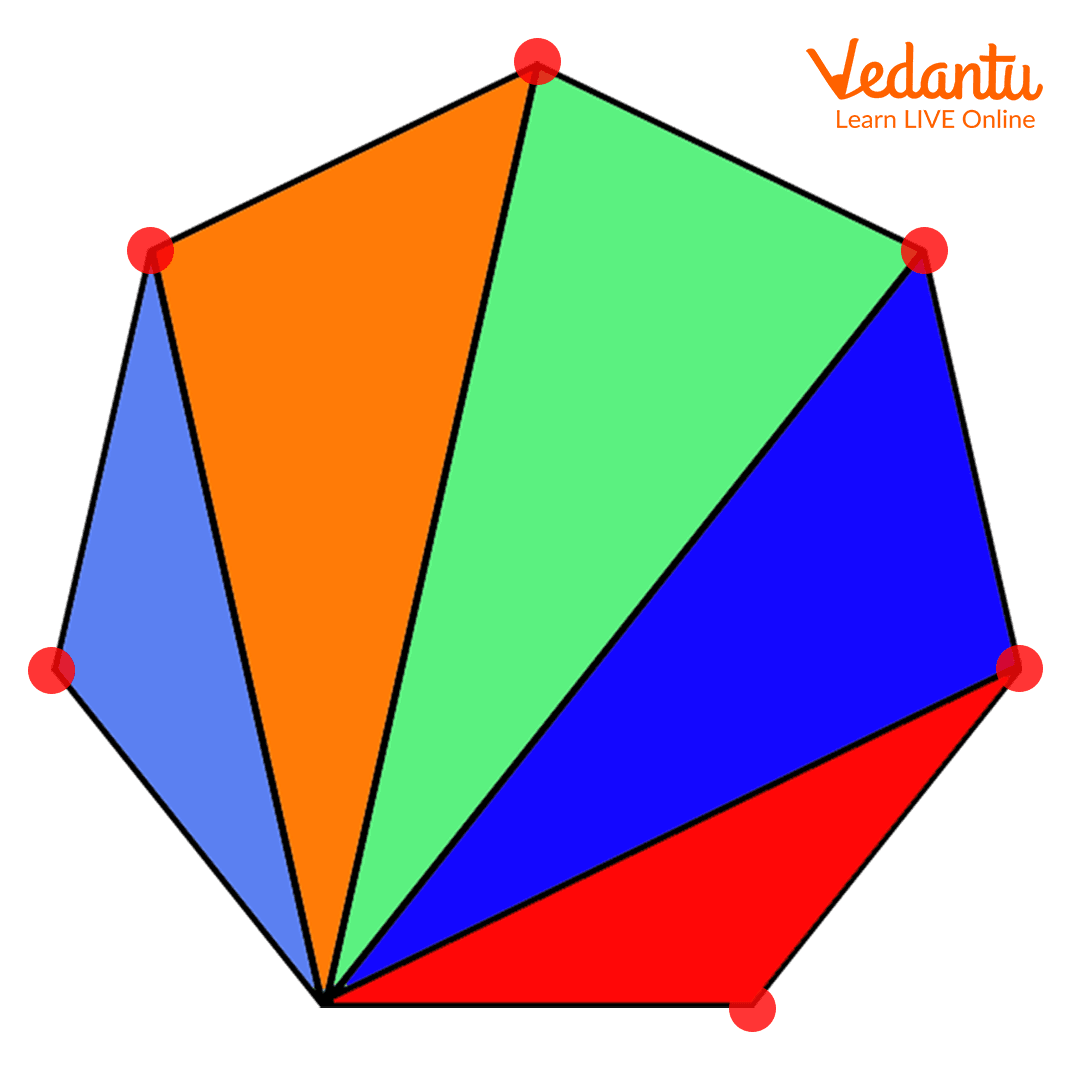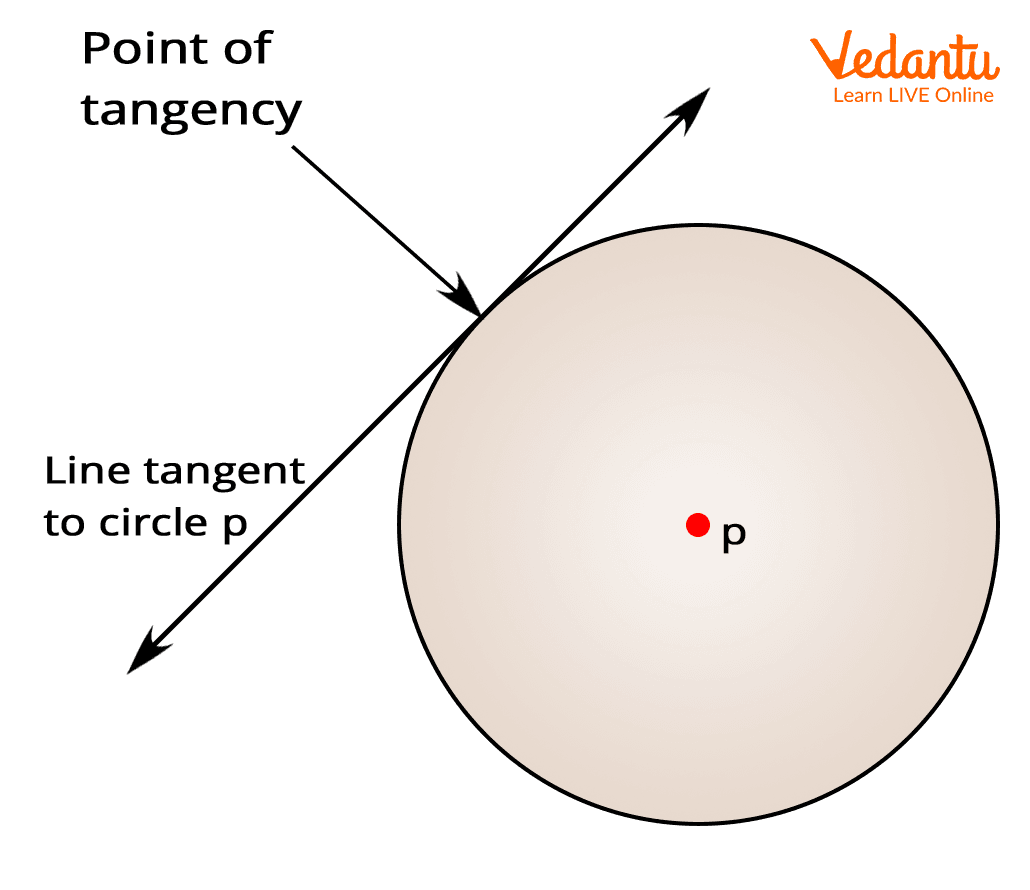Courses
Courses for Kids
Free study material
Free LIVE classes
MoreDo you know the counting? Count the numbers from 1 to 10…One, Two, Three, Four, Five, Six, Seven, Eight, Nine, Ten. Good! Can you tell the numbers that started with the alphabet ‘T’? ‘Two’, ‘Three’ and ‘Ten’ are the numbers that start with the letter ‘T’.  Very Good! There are many other Mathematical words that start with the letter ‘T’.

## Mathematics Vocabulary of Letter ‘T’

1. ‘T’ Numbers: Two, three, ten, twenty, thirty etc., are the numbers that start with the alphabet ‘T’. T for Trillion is considered as one of the largest numbers.

2. ‘T’ Places: Tens, Tenth, Thousand, Thousandth, Trillion, Trillionth are the places in the numbers system. These are necessary to form a number.3. ‘T’ Figures:  T for Triangle is the most common figure that starts from the letter T which has three sides. Tetrahedron, trapezoid or trapezium are some of the other figures that start from the letter ‘T’.

4. Table: In mathematics, T for ‘Table’ is a list of facts or figures called data that is usually arranged in rows and columns.

5. Trigonometry: T for trigonometry is a branch of mathematics that deals with the study of the triangle, its sides, angles or other parameters. Some other words related to trigonometry that start with ‘T’ are ‘Trigonometric Ratio’.

6. Division with ‘T’ Words: T for Trisect is a mathematical term that means the division of something into three equal parts.

Truncate is a word that is used to cut off the part of a number. For example, if 12.666666666666666 is truncated to the hundredth place then the number would be 12.66.

Triangulate means to split a polygon into non-overlapping triangles.Transversal is a line that intersects two or more lines.

7. Truth Value: It is generally used in boolean algebra to define any logic. It is represented by either 0 or 1.

8. Tangent: T for Tangent is defined as the line segment that intersects the curve or a circle at only one point.9. Terms:  In Algebra, a branch of Mathematics T for Terms is used to denote the parts of any equation. For example, in the equation 2xy + 7x + 9y three ‘terms’ are included.

10. Theorem: T for Theorem for any formula, proposition, statement or postulate that is deduced or to be deduced using other formulas, propositions or postulates.

## Do You Know?

• ‘Time’ is also considered as a mathematical word when it is used in reference to measuring the period, date or the moment when something happens.

• ‘Take away’ which means the process of subtracting something is a mathematical word that starts with the letter ‘T’.

## Conclusion

Here, you have added some key terms of mathematics in your pocket. These key terms start from the letter ‘T’. To add more such key terms that start from different alphabets, explore our website.

Last updated date: 21st Sep 2023
Total views: 137.1k
Views today: 2.37k